Test: Three Phase Induction Machine of Electrical Machines

# Test: Three Phase Induction Machine of Electrical Machines

Test Description

## 10 Questions MCQ Test GATE Electrical Engineering (EE) 2023 Mock Test Series | Test: Three Phase Induction Machine of Electrical Machines

Test: Three Phase Induction Machine of Electrical Machines for Electrical Engineering (EE) 2023 is part of GATE Electrical Engineering (EE) 2023 Mock Test Series preparation. The Test: Three Phase Induction Machine of Electrical Machines questions and answers have been prepared according to the Electrical Engineering (EE) exam syllabus.The Test: Three Phase Induction Machine of Electrical Machines MCQs are made for Electrical Engineering (EE) 2023 Exam. Find important definitions, questions, notes, meanings, examples, exercises, MCQs and online tests for Test: Three Phase Induction Machine of Electrical Machines below.
Solutions of Test: Three Phase Induction Machine of Electrical Machines questions in English are available as part of our GATE Electrical Engineering (EE) 2023 Mock Test Series for Electrical Engineering (EE) & Test: Three Phase Induction Machine of Electrical Machines solutions in Hindi for GATE Electrical Engineering (EE) 2023 Mock Test Series course. Download more important topics, notes, lectures and mock test series for Electrical Engineering (EE) Exam by signing up for free. Attempt Test: Three Phase Induction Machine of Electrical Machines | 10 questions in 30 minutes | Mock test for Electrical Engineering (EE) preparation | Free important questions MCQ to study GATE Electrical Engineering (EE) 2023 Mock Test Series for Electrical Engineering (EE) Exam | Download free PDF with solutions
 1 Crore+ students have signed up on EduRev. Have you?
Test: Three Phase Induction Machine of Electrical Machines - Question 1

### A 3-phase, 50 Hz induction motor has a full load speed of 1440 rpm. The number of poles of the motor is

Detailed Solution for Test: Three Phase Induction Machine of Electrical Machines - Question 1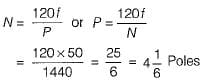Since the number of poles must be even and a whole number, therefore the number of poles must be 4.

Test: Three Phase Induction Machine of Electrical Machines - Question 2

### A properly shunted centre-zero galvanometer is connected in the rotor circuit of a 6-pole, 50 Hz would-rotor induction motor. If the galvanometer makes 90 complete oscillations in one minute, the speed of rotor would be

Detailed Solution for Test: Three Phase Induction Machine of Electrical Machines - Question 2

Rotor frequency,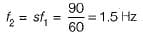∴ Slip,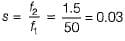So, rotor speed,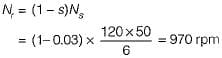Test: Three Phase Induction Machine of Electrical Machines - Question 3

### A 10-pole, 3-phase, 50 Hz, delta connected induction motor takes 120 kW during blocked-rotor test at rated voltage and frequency. If the stator ohmic loss is equal to rotor ohmic loss during blocked rotor test, then the starting torque if DOL starting is used for this motor will be

Detailed Solution for Test: Three Phase Induction Machine of Electrical Machines - Question 3

During blocked rotor test, power input to a 3-phase induction motor appears almost entirely as I2R loss in both stator and rotor windings. Since, stator ohmic loss = rotor ohmic loss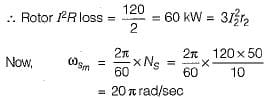So, starting torque,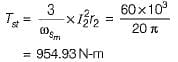Test: Three Phase Induction Machine of Electrical Machines - Question 4

A 3-phase squirrel cage induction motor has maximum torque equal to twice the full-load torque. The per phase rotor resistance and per phase standstill reactance referred to stator are 0.2 Ω and 2 Ω respectively (Stator impedance is neglected). What is the ratio of motor starting toque to its full-load torque, if the motor is started by direct-on-line starter?

Detailed Solution for Test: Three Phase Induction Machine of Electrical Machines - Question 4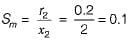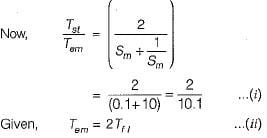From equations (i) and (ii), we have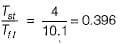Test: Three Phase Induction Machine of Electrical Machines - Question 5

A 10 kW, 400 V, 3-phase induction motor draw a current of 5 times its full-load current at rated voltage and at standstill developing a starting toque of 1.5 times its full-load torque. An autotransformer is installed to reduce the starting current and to give full-load torque at starting. Neglecting exciting current of auto-transformer, the voltage applied to the motor terminals at the time of starting with auto transformer is

Detailed Solution for Test: Three Phase Induction Machine of Electrical Machines - Question 5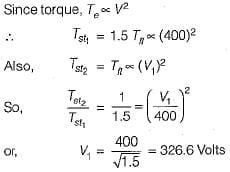Test: Three Phase Induction Machine of Electrical Machines - Question 6

A 3-phase, 20 kW, 400 V, 1470 rpm, 50 Hz squirrel cage induction motor develops a torque of 100 N-m at a speed of 1400 rpm. if the motor is connected to a 30 Hz supply; for keeping the same air-gap flux, the supply voltage and the speed for the same load torque would respectively be

Detailed Solution for Test: Three Phase Induction Machine of Electrical Machines - Question 6

For same air-gap flux,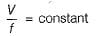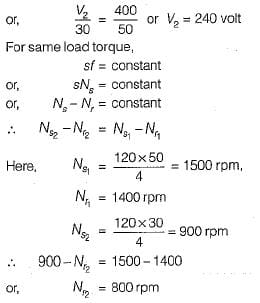Test: Three Phase Induction Machine of Electrical Machines - Question 7

In a 3-phase squirrel cage induction motor, applied voltage is reduced to x times its rated value. Then, its starting line current from mains and starting torque by auto-transformer starting would reduce respectively from their corresponding direct switching values by a factor of

Test: Three Phase Induction Machine of Electrical Machines - Question 8

A 3-phase IM with stator in delta draws a line current of 10 A. When connected in star, it would draw a line current of

Detailed Solution for Test: Three Phase Induction Machine of Electrical Machines - Question 8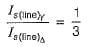or,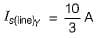Test: Three Phase Induction Machine of Electrical Machines - Question 9

The power factor of a delta-connected 3-phase, 50 kW, IM is 0.4 when delivering 35% of its rated load. If the stator is reconnected in star, then its

Detailed Solution for Test: Three Phase Induction Machine of Electrical Machines - Question 9

We know that,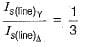∴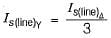Thus, stator current will decrease.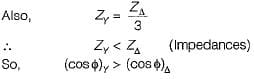Test: Three Phase Induction Machine of Electrical Machines - Question 10

A 3-phase, star-connected SRIM is fed from 400 V, 50 Hz source. Stator to rotor effective turns ratio is 2. At a rotor speed of 1440 rpm, the rotor induced emf per phase would be

Detailed Solution for Test: Three Phase Induction Machine of Electrical Machines - Question 10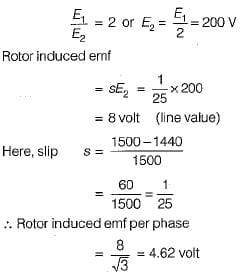## GATE Electrical Engineering (EE) 2023 Mock Test Series

22 docs|274 tests
Information about Test: Three Phase Induction Machine of Electrical Machines Page
In this test you can find the Exam questions for Test: Three Phase Induction Machine of Electrical Machines solved & explained in the simplest way possible. Besides giving Questions and answers for Test: Three Phase Induction Machine of Electrical Machines, EduRev gives you an ample number of Online tests for practice

## GATE Electrical Engineering (EE) 2023 Mock Test Series

22 docs|274 tests(Scan QR code)